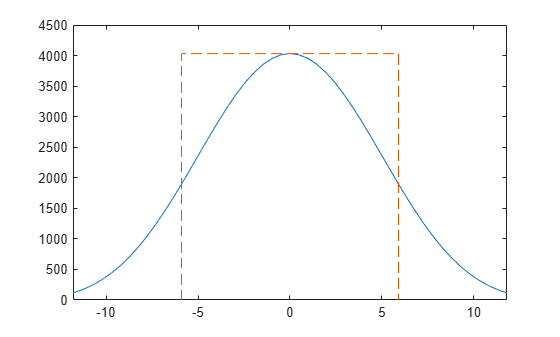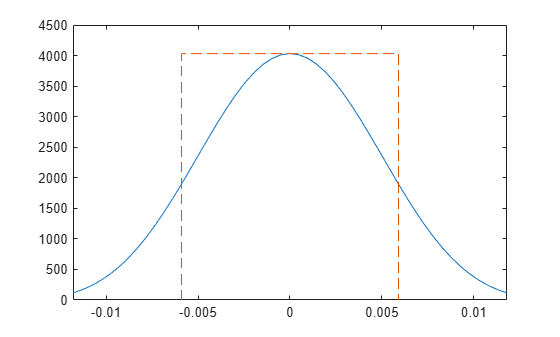# enbw

Equivalent noise bandwidth

## Syntax

``bw = enbw(window)``
``bw = enbw(window,fs)``

## Description

example

````bw = enbw(window)` returns the two-sided equivalent noise bandwidth, `bw`, for a uniformly sampled window, `window`. The equivalent noise bandwidth is normalized by the noise power per frequency bin.```

example

````bw = enbw(window,fs)` returns the two-sided equivalent noise bandwidth, `bw`, in Hz.```

## Examples

collapse all

Determine the equivalent noise bandwidth of a Hamming window 1000 samples in length.

`bw = enbw(hamming(1000))`
```bw = 1.3638 ```

Determine the equivalent noise bandwidth in Hz of a flat top window 10000 samples in length. The sample rate is 44.1 kHz.

`bw = enbw(flattopwin(10000),44.1e3)`
```bw = 16.6285 ```

Obtain the equivalent rectangular noise bandwidth of a 128-sample Hann window.

Generate the window and compute its discrete Fourier transform over 2048 frequencies. Shift the transform so it is centered at zero frequency and compute its squared magnitude.

```lw = 128; win = hann(lw); lt = 2048; windft = fftshift(fft(win,lt)); ad = abs(windft).^2; mg = max(ad);```

Specify a sample rate of 1 kHz. Use `enbw` to compute the equivalent noise bandwidth of the window and verify that the value coincides with the definition.

```fs = 1000; bw = enbw(win,fs)```
```bw = 11.8110 ```
`bdef = sum((win).^2)/sum(win)^2*fs`
```bdef = 11.8110 ```

Plot the squared magnitude of the window. Overlay a rectangle with height equal to the peak of the squared magnitude and width equal to the equivalent noise bandwidth.

```freq = -fs/2:fs/lt:fs/2-fs/lt; plot(freq,ad, ... bw/2*[-1 -1 1 1],mg*[0 1 1 0],'--') xlim(bw*[-1 1])```Verify that the area of the rectangle contains the same total power as the window.

`Adiff = trapz(freq,ad)-bw*mg`
```Adiff = 1.4552e-11 ```

Repeat the computation using normalized frequencies. Find the equivalent noise bandwidth of the window. Verify that `enbw` gives the same value as the definition.

`bw = enbw(win)`
```bw = 1.5118 ```
`bdef = sum((win).^2)/sum(win)^2*lw`
```bdef = 1.5118 ```

Plot the squared magnitude of the window. Overlay a rectangle with height equal to the peak of the squared magnitude and width equal to the equivalent noise bandwidth.

```freqn = -1/2:1/lt:1/2-1/lt; plot(freqn,ad, ... bw/2*[-1 -1 1 1]/lw,mg*[0 1 1 0],'--') xlim(bw*[-1 1]/lw)```Verify that the area of the rectangle contains the same total power as the window.

`Adiff = trapz(freqn,ad)-bw*mg/lw`
```Adiff = 0 ```

## Input Arguments

collapse all

Uniformly sampled window vector, specified as a row or column vector with real-valued elements.

Example: `hamming(1000)`

Data Types: `double` | `single`

Sampling frequency, specified as a positive scalar.

## Output Arguments

collapse all

Equivalent noise bandwidth, specified as a positive scalar.

Data Types: `double` | `single`

collapse all

### Equivalent Noise Bandwidth

The total power contained in a window is the area under the curve produced by the squared magnitude of the Fourier transform of the window. The equivalent noise bandwidth of the window is the width of a rectangle with height equal to the peak of the squared magnitude of the Fourier transform and area equal to the total power.

Parseval's theorem states that the total energy for the N-sample window w(n) equals the sum of the squared magnitudes of the time-domain window samples or the integral over frequency of the squared magnitude of the Fourier transform of the window:

`${\int }_{-1/2}^{1/2}{|W\left(f\right)|}^{2}df=\sum _{n}{|w\left(n\right)|}^{2}.$`

The power spectrum of the window has peak magnitude at f = 0:

`${|W\left(0\right)|}^{2}={|\sum _{n}w\left(n\right)|}^{2}.$`

To find the width of the equivalent rectangle, and thus the equivalent noise bandwidth, divide the area by the height:

`$\frac{{\int }_{-1/2}^{1/2}{|W\left(f\right)|}^{2}df}{{|W\left(0\right)|}^{2}}=\frac{\sum _{n}{|w\left(n\right)|}^{2}}{{|\sum _{n}w\left(n\right)|}^{2}}.$`

When a sample rate fs is specified, `enbw` returns the previous expression multiplied by fs:

`$\text{ENBW}={f}_{s}\frac{\sum _{n}{|w\left(n\right)|}^{2}}{{|\sum _{n}w\left(n\right)|}^{2}}.$`

For normalized frequencies, the function divides by the bin width, fs/N:

`$\text{ENBW}=N\frac{\sum _{n}{|w\left(n\right)|}^{2}}{{|\sum _{n}w\left(n\right)|}^{2}}.$`

See Equivalent Rectangular Noise Bandwidth for an example that, for a given window:

• Compares the result of `enbw` to the definition for a specified sample rate and for normalized frequencies.

• Plots the equivalent rectangular bandwidth over the magnitude spectrum of the window.

• Verifies that the total power contained in the window is the same as the power contained in a rectangle with height equal to the peak squared magnitude of the Fourier transform of the window and width equal to the equivalent noise bandwidth.

 harris, fredric j. “On the Use of Windows for Harmonic Analysis with the Discrete Fourier Transform.” Proceedings of the IEEE®. Vol. 66, January 1978, pp. 51–83.